# LeetCode周赛311，中规中矩的裸题大赛……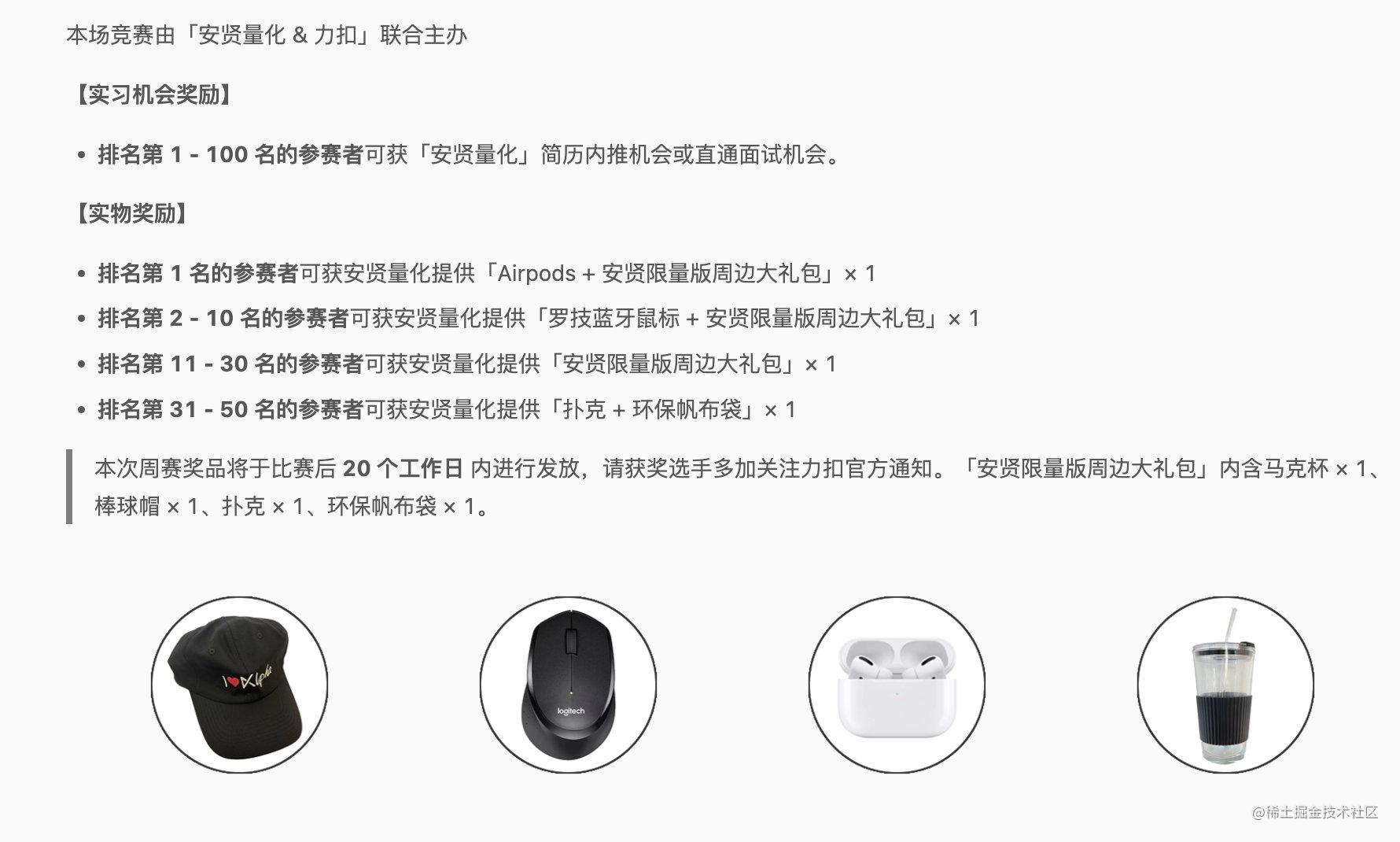## 最小偶倍数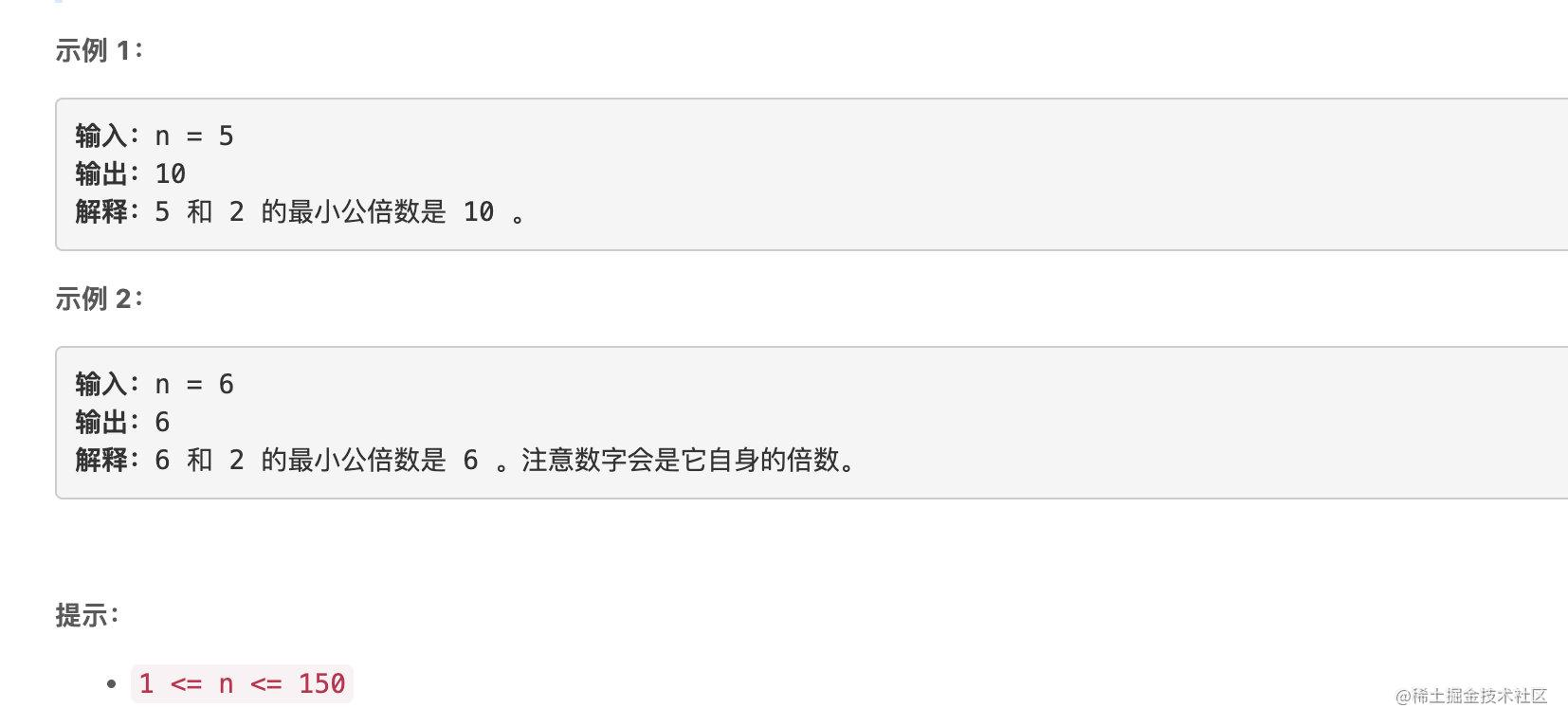### 题解

``````class Solution {
public:
int smallestEvenMultiple(int n) {
return n % 2 ? n << 1: n;
}
};

## 最长的字母序连续子字符串的长度

• 例如，`"abc"` 是一个字母序连续字符串，而 `"acb"``"za"` 不是。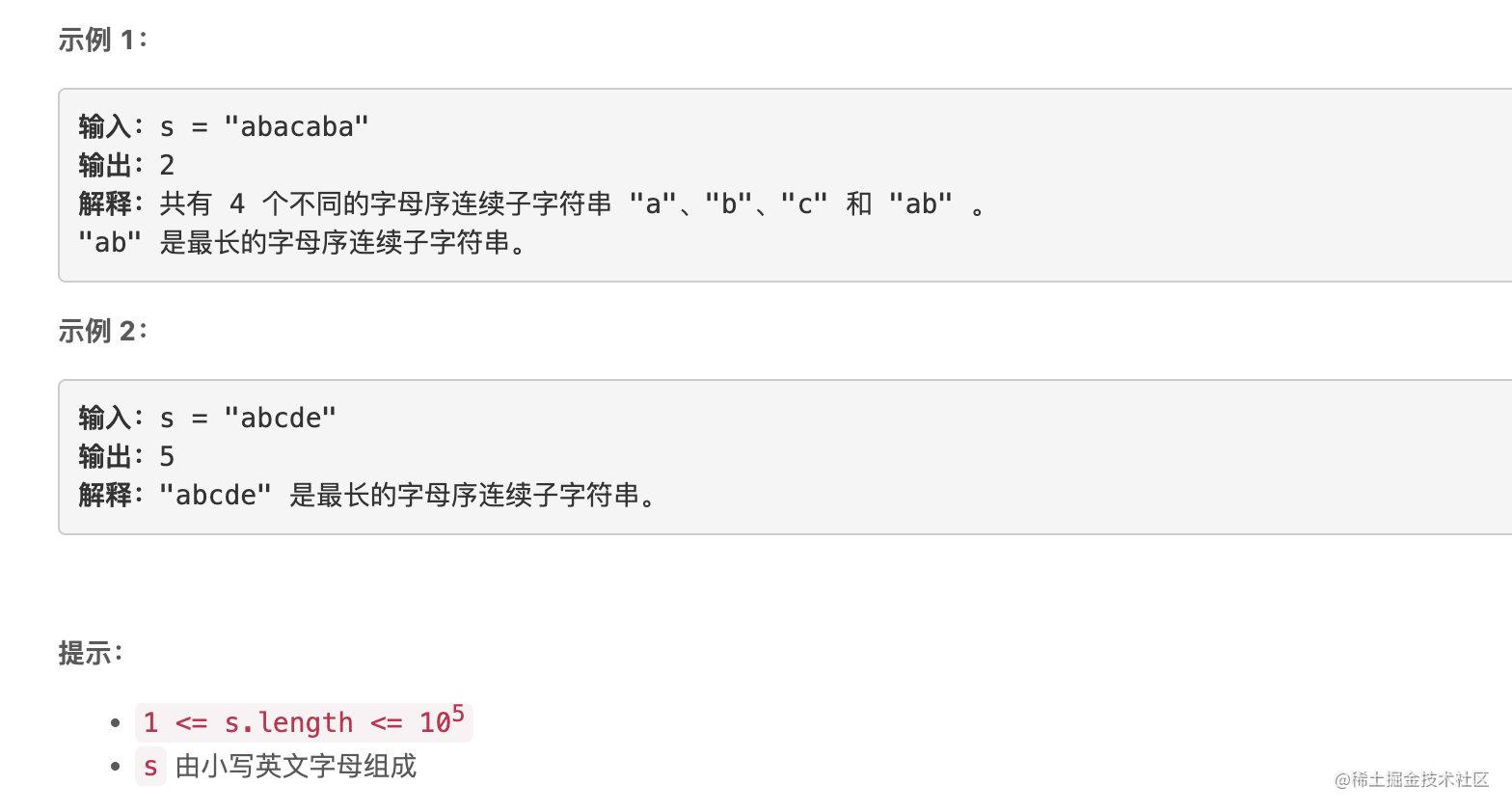### 题解

two pointer裸题。

``````class Solution {
public:
int longestContinuousSubstring(string s) {
int l = 0;

int ret = 1;
for (int r = 1; r < s.length(); r++) {
if (s[r] != s[r-1]+1) {
l = r;
}
ret = max(r - l + 1, ret);
}
return ret;
}
};

## 反转二叉树的奇数层

• 例如，假设第 3 层的节点值是 `[2,1,3,4,7,11,29,18]` ，那么反转后它应该变成 `[18,29,11,7,4,3,1,2]`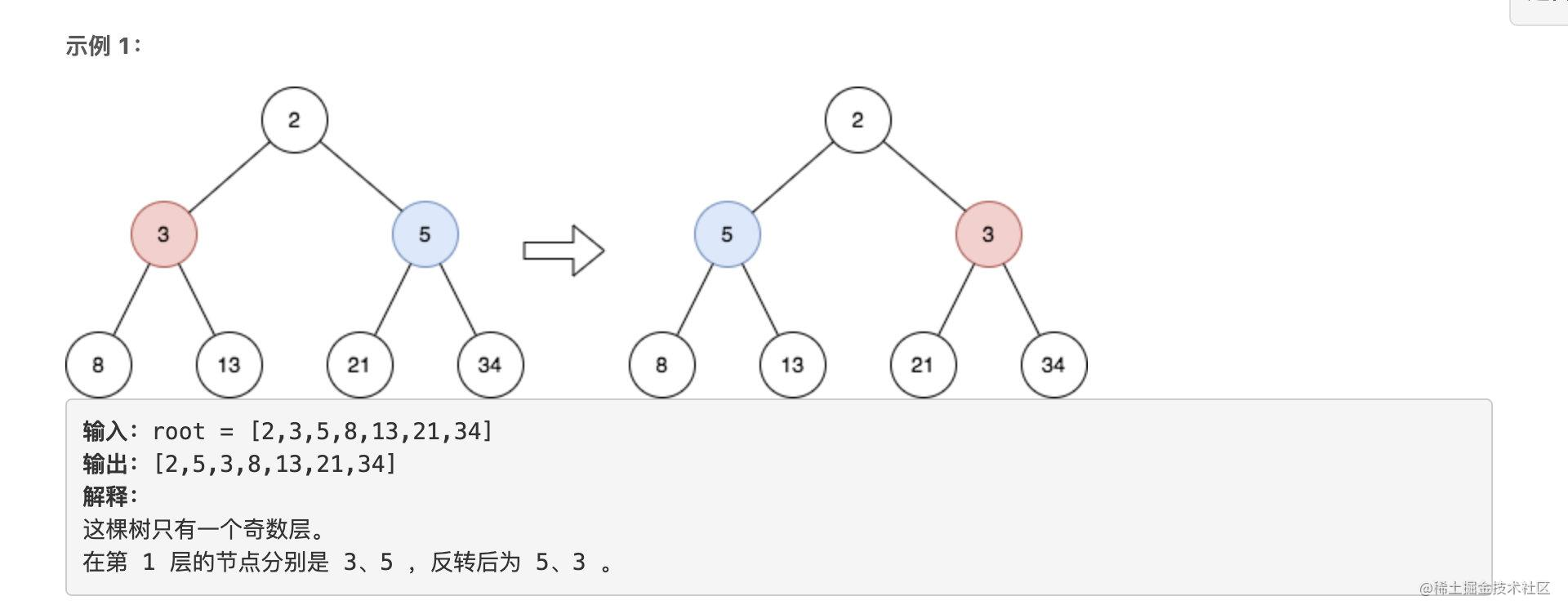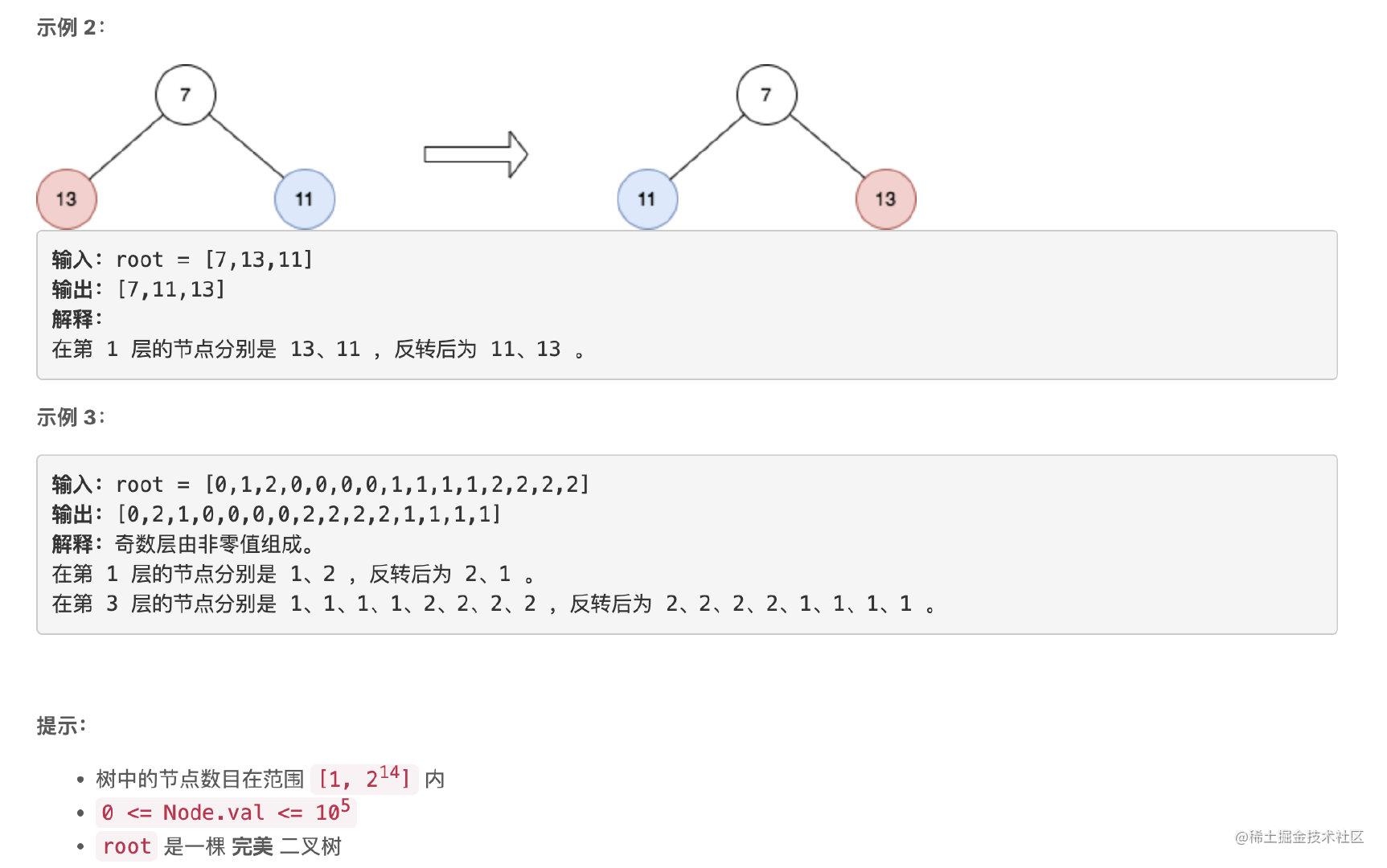### 题解

``````/**
* Definition for a binary tree node.
* struct TreeNode {
*     int val;
*     TreeNode *left;
*     TreeNode *right;
*     TreeNode() : val(0), left(nullptr), right(nullptr) {}
*     TreeNode(int x) : val(x), left(nullptr), right(nullptr) {}
*     TreeNode(int x, TreeNode *left, TreeNode *right) : val(x), left(left), right(right) {}
* };
*/
class Solution {
public:
TreeNode* reverseOddLevels(TreeNode* root) {
// cur存储当前层的节点，next存储下一层
vector<TreeNode*> cur, next;
// 记录下一层是否要翻转
bool flag = true;

cur.push_back(root);
while (!cur.empty()) {
for (auto &u: cur) {
if (u->left != nullptr) {
next.push_back(u->left);
next.push_back(u->right);
}
}

if (flag) {
int l = 0, r = next.size() - 1;
while (l < r) {
swap(next[l]->val, next[r]->val);
l++;
r--;
}
}
flag = !flag;
cur = next;
next = vector<TreeNode*> ();
}
return root;
}
};

## 字符串的前缀分数和

• 例如，如果 `words = ["a", "ab", "abc", "cab"]` ，那么 `"ab"` 的分数是 `2` ，因为 `"ab"``"ab"``"abc"` 的一个前缀。

**注意：**字符串视作它自身的一个前缀。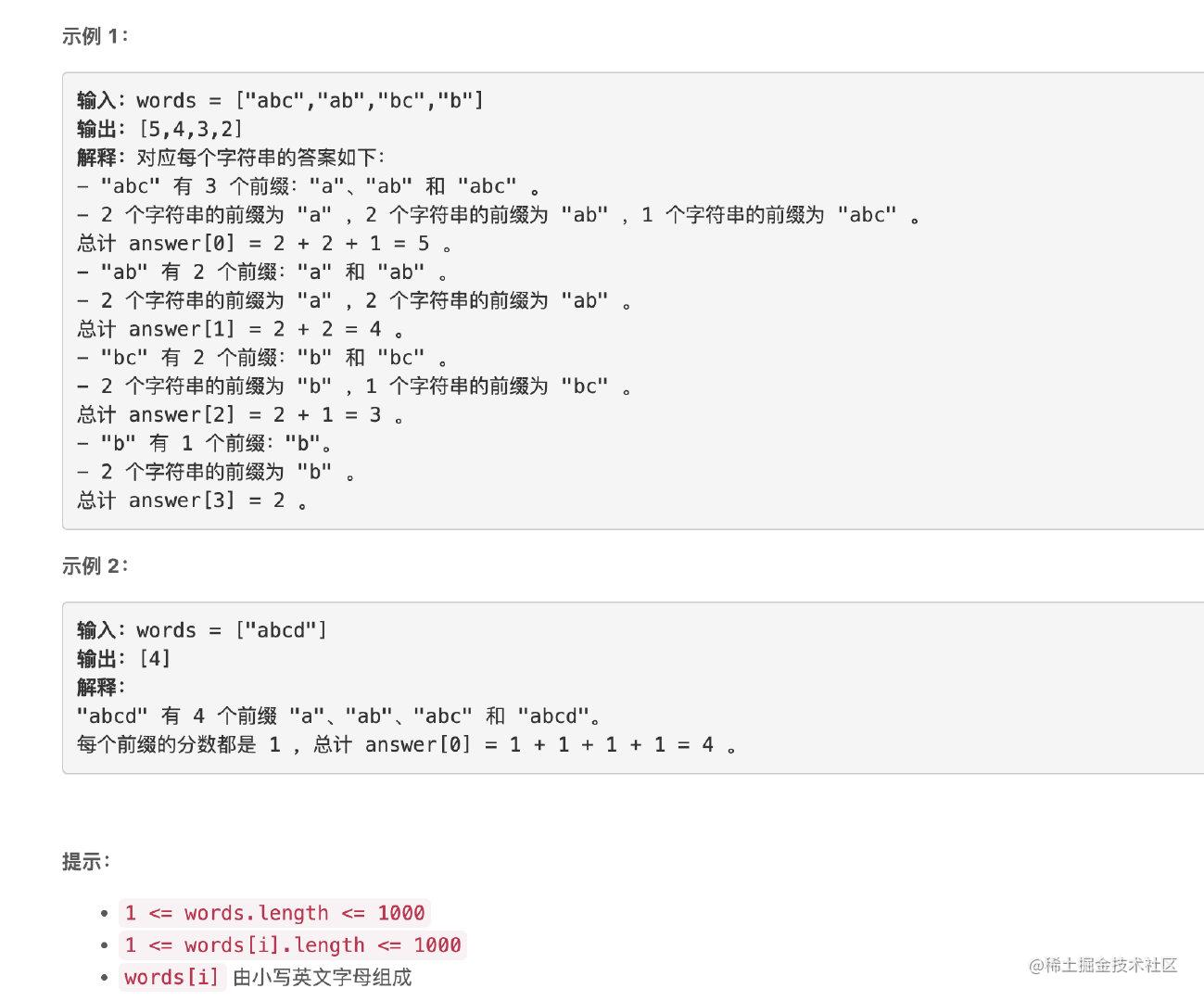### 题解

``````class Solution {
public:

struct Node {
int v;
Node* chs = {nullptr};
Node(): v(1) {};
};

struct Trie {
Node *node;
Trie() {
node = new Node();
}
void insert(string &str) {
Node *cur = node;
for (auto c: str) {
int idx = c - 'a';
if (cur->chs[idx] == nullptr) {
cur->chs[idx] = new Node();
}else {
cur->chs[idx]->v++;
}
cur = cur->chs[idx];
}
}
int query(string &str) {
int ret = 0;
Node *cur = node;
for (auto c: str) {
int idx = c - 'a';
if (cur->chs[idx] == nullptr) break;
ret += cur->chs[idx]->v;
cur = cur->chs[idx];
}
return ret;
}
};

vector<int> sumPrefixScores(vector<string>& words) {
vector<int> ret;
Trie* t = new Trie();
for (auto &st : words) {
t->insert(st);
}

for (auto &st: words) {
int cur = t->query(st);
ret.push_back(cur);
}
return ret;
}
};